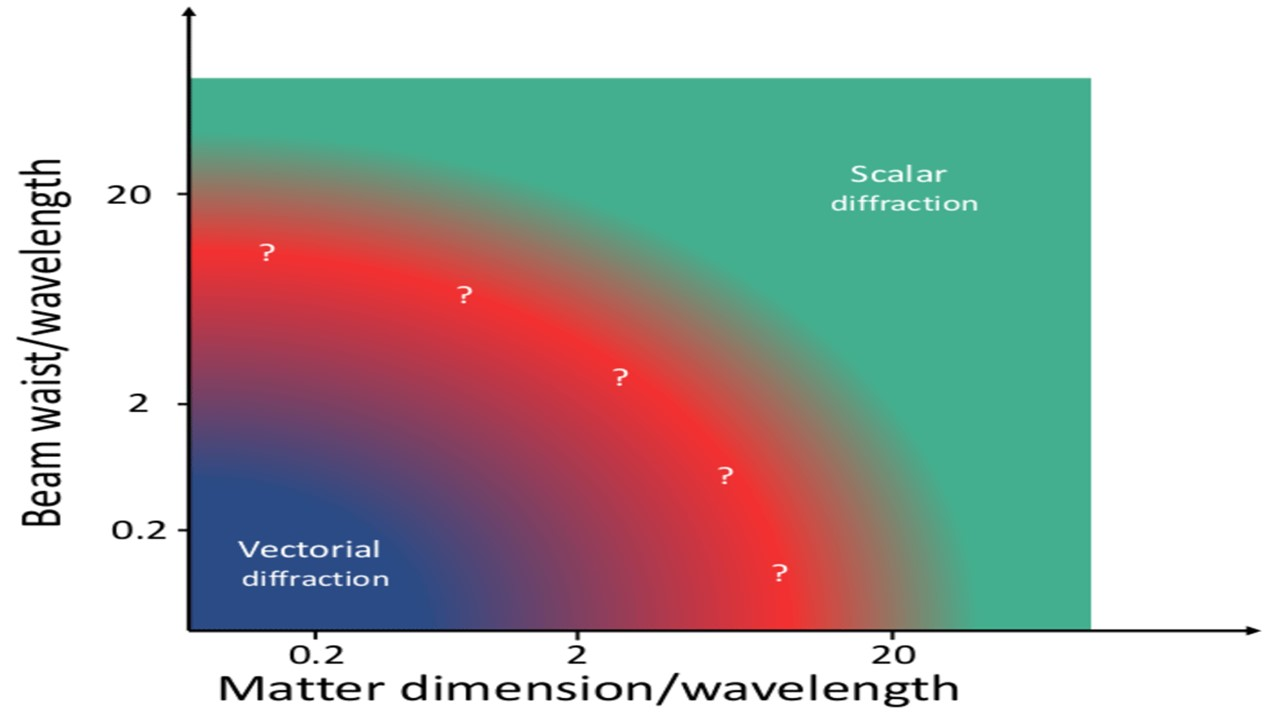# An experimental technique to assess the scalar vectorial regime of diffraction

#### Background:

In classical electrodynamics, light-matter interactions are modelled using Maxwell equations. The solution of Maxwell equations, which is typically given by means of the electric and magnetic field, is vectorial in nature. Yet it is well known that light-matter interactions can be approximately described in a scalar (polarization independent) way for many optical applications. While the accuracy of the scalar approximation can be theoretically computed, to the best of our knowledge, it has never been determined experimentally. Here, we show that the vectoriality of diffraction can be probed with a new technique: Vortex Circular Dichroism(VCD).